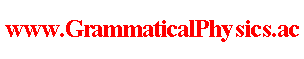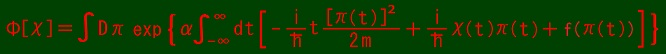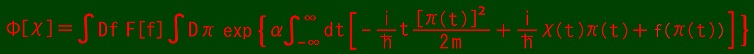since 2006 Help　Sitemap < Forum > < Products > < Quantum History Theory > < Exact Solution of Uda Equation (6) > In this page, I write exact solutions of Uda Equation in case of V = 0.. where f: R → C. Φ[χ(□ - ε)] = ∫Dπ exp{α∫-∞∞dt[-(i/h)tπ(t)2/(2m) + f(π(t))]} exp[(i/h)α∫-∞∞dt'π(t')χ(t' - ε)] 　= ∫Dπ exp{α∫-∞∞dt[-(i/h)tπ(t)2/(2m) + f(π(t))]} exp[(i/h)α∫-∞∞dt'π(t' + ε)χ(t')] 　= ∫Dπ' exp{α∫-∞∞dt[-(i/h)tπ'(t - ε)2/(2m) + f(π'(t - ε))]} exp[(i/h)α∫-∞∞dt'π'(t')χ(t')] 　= ∫Dπ' exp{α∫-∞∞dt[-(i/h)(t + ε)π'(t)2/(2m) + f(π'(t))]} exp[(i/h)α∫-∞∞dt'π'(t')χ(t')] where π'(t) = π(t + ε). ih limε→0 (1/ε) {Φ[χ(□ - ε)] - Φ[χ]} 　= ∫Dπ' {α∫-∞∞dt[π'(t)2/(2m)]} exp{α∫-∞∞dt[-(i/h)(t + ε)π'(t)2/(2m) + f(π'(t))]} exp[(i/h)α∫-∞∞dt'π'(t')χ(t')]. {α∫-∞∞dt[-(ih/α)δ/δχ(t)]2/(2m)]}Φ[χ] 　= ∫Dπ' {α∫-∞∞dt[π(t)2/(2m)]} exp{α∫-∞∞dt[-(i/h)tπ(t)2/(2m) + f(π(t))]} exp[(i/h)α∫-∞∞dt'π(t')χ(t')]. ∴ ih limε→0 (1/ε) {Φ[χ(□ - ε)] - Φ[χ]} = {α∫-∞∞dt[-(ih/α)δ/δχ(t)]2/(2m)]}Φ[χ]. ∫-∞∞dp exp{α∫aa +εdt[-(i/h)tp2/(2m) + (i/h)xp + f(p)]} (ε→ +0) 　=∫-∞∞dp exp{αε[-(i/h)ap2/(2m) + (i/h)xp + f(p)]} 　= exp{αεf([-ih/(αε)]∂/∂x)} ∫-∞∞dp exp{αε[-(i/h)ap2/(2m) + (i/h)xp]} 　= exp{αεf([-ih/(αε)]∂/∂x)} √{π/[αε(i/h)a/(2m)]}・exp{(-1/h2)α2ε2x2/[4αε(i/h)a/(2m)]} 　= √[2mhπ/(iαεa)]・exp{αεf([-ih/(αε)]∂/∂x)} exp{[imαε/(2ha)]x2}. If f(p) = 0, ψ(x, t) = exp{[im/(2h)](x2/t)}. If f(p) = kp, ∫-∞∞dp exp{αε[-(i/h)ap2/(2m) + (i/h)xp + f(p)]} = ∫-∞∞dp exp[αε{-(i/h)ap2/(2m) + [(i/h)x + k]p}] = c exp{αε[(i/h)x + k]2/[4(i/h)a/(2m)]} = c exp{αε[im/(2h)](x - ihk)2/a} ∴ ∫Dπ exp{α∫aa+1/αdt[-(i/h)tπ(t)2/(2m) + (i/h)xπ(t) + f(π(t))]} 　　≒ c'[∫-∞∞dp exp{αε[-(i/h)ap2/(2m) + (i/h)xp + f(p)]}]1/(αε) 　　= c''exp{[im/(2h)](x - ihk)2/a}. If f(p) = -K(p - b)2, ∫-∞∞dp exp{αε[-(i/h)ap2/(2m) + (i/h)xp + f(p)]} = ∫-∞∞dp exp{-αε[K + (i/h)a/(2m)]p2 + αε[(i/h)x + 2bK]p - αεKb2} = C exp{αε(1/4)[(i/h)x + 2bK]2/[K + (i/h)a/(2m)]} = C exp{αε(-1/2)(m/h)(x - 2ihbK)2/(2hmK + ia)} ∴ ∫Dπ exp{α∫aa+1/αdt[-(i/h)tπ(t)2/(2m) + (i/h)xπ(t) + f(π(t))]} 　　≒ C'[∫-∞∞dp exp{αε[-(i/h)ap2/(2m) + (i/h)xp + f(p)]}]1/(αε) 　　= C''exp{-(1/2)(m/h)(x - 2ihbK)2/(2hmK + ia)}. I can not calculate for general f but I assume that the following functional integral converges except for constant factor for wide variety of f.∫Dπ exp{α∫aa+1/αdt[-(i/h)tπ(t)2/(2m) + (i/h)xπ(t) + f(π(t))]}. Any superposition of solutions of the form at the top of this page is also a solution. It is as follows.where F is an arbitrary complex valued functional. This perhaps covers all general solutions. --- The original form of the solution shown in this page started being displayed at the top of this page at 2019/11/11/21:05JST. It was of the same meaning as the meaning of the present form though it was transformed into the present form later. Gaussian integration was done on 2019/11/15. Author Yuichi Uda, Write start at 2019/11/11/20:15JST, Last edit at 2019/12/06/17:13JST